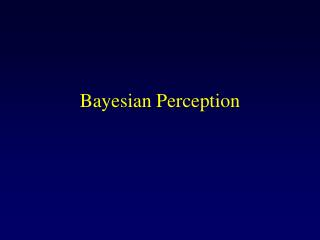# Bayesian Perception - PowerPoint PPT PresentationDownload PresentationBayesian Perception

Bayesian PerceptionDownload Presentation## Bayesian Perception

- - - - - - - - - - - - - - - - - - - - - - - - - - - E N D - - - - - - - - - - - - - - - - - - - - - - - - - - -
##### Presentation Transcript

1. Bayesian Perception

2. General Idea Ernst and Banks, Nature, 2002

3. General Idea • Bayesian formulation: Conditional Independence assumption

4. noise v=w+n + w t=w+n + noise General Idea Generative model: w? Ernst and Banks, Nature, 2002

5. Bimodal P(w|t,v)= aP(v|w)P(t|w) Visual P(v|w) Touch P(t|w) General Idea Probability Width

6. General Idea

7. General Idea Mean and variance

8. Visual P(v|w) Touch P(t|w) General Idea Probability Width v t

9. General Idea Mean and variance

10. Optimal Variance Variance Fisher information sums for independent signals

11. Unimodal Tactile STD Unimodal visual STD Measured bimodal STD General Idea Predicted by the Bayesian model 0.2 0.15 Threshold (STD) 0.1 0.05 0 0 67 133 200 Visual noise level (%) Note: unimodal estimates may not be optimal but the multimodal estimate is optimal Ernst and Banks, Nature, 2002

12. Adaptive Cue Integration • Note: the reliability of the cue change on every trial • This implies that the weights of the linear combination have to be changed on every trial! • Or do they?

13. General Idea • Perception is a statistical inference • The brain stores knowledge about P(I,V) where I is the set of natural images, and V are the perceptual variables (color, motion, object identity) • Given an image, the brain computes P(V|I)

14. General Idea • Decisions are made by collapsing the distribution onto a single value: • or

15. Key Ideas • The nervous systems represents probability distributions. i.e., it represents the uncertainty inherent to all stimuli. • The nervous system stores generative models, or forward models, of the world (P(I|V)), and prior knowlege about the state of the world (P(V)) • Biological neural networks can perform complex statistical inferences.

16. Motion Perception

17. The Aperture Problem

18. The Aperture Problem

19. The Aperture Problem

20. The Aperture Problem Vertical velocity (deg/s) Horizontal velocity (deg/s)

21. The Aperture Problem Vertical velocity (deg/s) Horizontal velocity (deg/s)

22. The Aperture Problem

23. The Aperture Problem Vertical velocity (deg/s) Horizontal velocity (deg/s)

24. The Aperture Problem Vertical velocity (deg/s) Horizontal velocity (deg/s)

25. The Aperture Problem Vertical velocity (deg/s) Horizontal velocity (deg/s)

26. Standard Models of Motion Perception • IOC: interception of constraints • VA: Vector average • Feature tracking

27. Standard Models of Motion Perception IOC VA Vertical velocity (deg/s) Horizontal velocity (deg/s)

28. Standard Models of Motion Perception IOC VA Vertical velocity (deg/s) Horizontal velocity (deg/s)

29. Standard Models of Motion Perception IOC VA Vertical velocity (deg/s) Horizontal velocity (deg/s)

30. Standard Models of Motion Perception IOC VA Vertical velocity (deg/s) Horizontal velocity (deg/s)

31. Standard Models of Motion Perception • Problem: perceived motion is close to either IOC or VA depending on stimulus duration, eccentricity, contrast and other factors.

32. Standard Models of Motion Perception • Example: Rhombus Percept: IOC Percept: VA IOC IOC VA VA Vertical velocity (deg/s) Vertical velocity (deg/s) Horizontal velocity (deg/s) Horizontal velocity (deg/s)

33. Moving Rhombus

34. Bayesian Model of Motion Perception • Perceived motion correspond to the MAP estimate

35. 50 0 Vertical Velocity -50 -50 0 50 Horizontal Velocity Prior • Human observers favor slow motions

36. 50 Vertical Velocity 0 -50 -50 0 50 Horizontal Velocity Likelihood • Weiss and Adelson

37. Likelihood

38. Likelihood Binary maskPresumably, this is set by segmentation cues

39. Posterior

40. Bayesian Model of Motion Perception • Perceived motion corresponds to the MAP estimate Only one free parameter

41. Likelihood

42. Motion through an Aperture • Humans perceive the slowest motion. • More generally: we tend to perceive the most likely interpretation of an image

43. Motion through an Aperture Likelihood 50 Vertical Velocity 0 -50 -50 0 50 ML Horizontal Velocity 50 50 Vertical Velocity Vertical Velocity MAP 0 0 -50 -50 Prior Posterior -50 0 50 -50 0 50 Horizontal Velocity Horizontal Velocity

44. Motion and Constrast • Humans tend to underestimate velocity in low contrast situations

45. Motion and Contrast Likelihood 50 Vertical Velocity 0 -50 High Contrast -50 0 50 ML Horizontal Velocity 50 50 Vertical Velocity Vertical Velocity MAP 0 0 -50 -50 Prior Posterior -50 0 50 -50 0 50 Horizontal Velocity Horizontal Velocity

46. Motion and Contrast Likelihood 50 Vertical Velocity 0 -50 Low Contrast -50 0 50 ML Horizontal Velocity MAP 50 50 Vertical Velocity Vertical Velocity 0 0 -50 -50 Prior Posterior -50 0 50 -50 0 50 Horizontal Velocity Horizontal Velocity

47. Motion and Contrast • Driving in the fog: in low contrast situations, the prior dominates

48. Moving Rhombus Likelihood 50 50 Vertical Velocity Vertical Velocity 0 0 -50 -50 High Contrast -50 0 50 -50 0 50 IOC Horizontal Velocity Horizontal Velocity MAP 50 50 Vertical Velocity Vertical Velocity 0 0 -50 -50 -50 0 50 -50 0 50 Prior Posterior Horizontal Velocity Horizontal Velocity

49. Moving Rhombus Likelihood 50 50 Vertical Velocity Vertical Velocity 0 0 -50 -50 Low Contrast -50 0 50 -50 0 50 IOC Horizontal Velocity Horizontal Velocity 50 50 MAP Vertical Velocity Vertical Velocity 0 0 -50 -50 -50 0 50 -50 0 50 Prior Posterior Horizontal Velocity Horizontal Velocity

50. Moving Rhombus